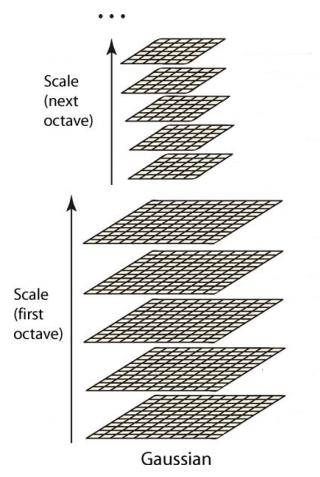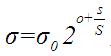•全国客户服务热线：
0755-23712116
•邮箱：
contact@shuangyi-tech.com# 高斯金字塔的构建步骤

SIFT(Scale-Invariant Feature Transform，尺度不变特征转换)在目标识别、图像配准领域具有广泛的应用，下面按照SIFT特征的算法流程对其进行简要介绍对SIFT特征做简要介绍。σ，2^(1/3)σ，    2^(2/3)σ，     2^(3/3)σ，    2^(4/3)σ，     2^(5/3)σ；

σ，2^(1/3)σ，    2^(2/3)σ，     2^(3/3)σ，   2^(4/3)σ。

2×σ，2×2^(1/3)σ，2×2^(2/3)σ，2×2^(3/3)σ，2×2^(4/3)σ，2×2^(5/3)σ；

2×σ，2×2^(1/3)σ，2×2^(2/3)σ，2×2^(3/3)σ，2×2^(4/3)σ。1. void SIFT::buildGaussianPyramid( const Mat& base, vector<Mat>& pyr, int nOctaves ) const
2. {
3.     vector<double> sig(nOctaveLayers + 3);
4.     pyr.resize(nOctaves*(nOctaveLayers + 3));
5.     // precompute Gaussian sigmas using the following formula:
6.     //  \sigma_{total}^2 = \sigma_{i}^2 + \sigma_{i-1}^2
7.     sig = sigma;
8.     double k = pow( 2., 1. / nOctaveLayers );
9.     for( int i = 1; i < nOctaveLayers + 3; i++ )
10.     {
11.         double sig_prev = pow(k, (double)(i-1))*sigma;
12.         double sig_total = sig_prev*k;
13.         sig[i] = std::sqrt(sig_total*sig_total - sig_prev*sig_prev);
14.     }
15.     for( int o = 0; o < nOctaves; o++ )
16.     {
17.         for( int i = 0; i < nOctaveLayers + 3; i++ )
18.         {
19.             Mat& dst = pyr[o*(nOctaveLayers + 3) + i];
20.             if( o == 0  &&  i == 0 )
21.                 dst = base;
22.             // base of new octave is halved image from end of previous octave
23.             else if( i == 0 )/*每一个八度中第一幅图像的确定过程*/
24.             {
25.                   const Mat& src = pyr[(o-1)*(nOctaveLayers + 3) + nOctaveLayers];
26.                   resize(src, dst, Size(src.cols/2, src.rows/2), 0, 0, INTER_NEAREST);
27.             }
28.            else
29.            {
30.                     const Mat& src = pyr[o*(nOctaveLayers + 3) + i-1];
31.                     GaussianBlur(src, dst, Size(), sig[i], sig[i]);
32.            }
33.         }
34.      }
35. }
36. void SIFT::buildDoGPyramid( const vector<Mat>& gpyr, vector<Mat>& dogpyr ) const
37. {
38.         int nOctaves = (int)gpyr.size()/(nOctaveLayers + 3);
39.         dogpyr.resize( nOctaves*(nOctaveLayers + 2) );
40.         for( int o = 0; o < nOctaves; o++ )
41.         {
42.                for( int i = 0; i < nOctaveLayers + 2; i++ )
43.                {
44.                      const Mat& src1 = gpyr[o*(nOctaveLayers + 3) + i];
45.                      const Mat& src2 = gpyr[o*(nOctaveLayers + 3) + i + 1];
46.                      Mat& dst = dogpyr[o*(nOctaveLayers + 2) + i];
47.                      subtract(src2, src1, dst, noArray(), DataType<sift_wt>::type);
48.                }
49.         }
50. }

• 0755-23712116
• 13822267203